Next: The Direct Current Generator Up: Magnetic Induction Previous: Eddy Currents

The Alternating Current Generator

An electric generator, or dynamo, is a device which converts mechanical energy into electrical energy. The simplest practical generator consists of a rectangular coil rotating in a uniform magnetic field. The magnetic field is usually supplied by a permanent magnet. This setup is illustrated in Fig. 38.Let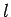be the length of the coil along its axis of rotation, and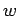the width of the coil perpendicular to this axis. Suppose that the coil rotates with constant angular velocityin a uniform magnetic field of strength. The velocitywith which the the two long sides of the coil (i.e., sides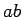and) move through the magnetic field is simply the product of the angular velocity of rotationand the distance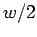of each side from the axis of rotation, so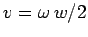. The motional emf induced in each side is given by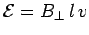, whereis the component of the magnetic field perpendicular to instantaneous direction of motion of the side in question. If the direction of the magnetic field subtends an anglewith the normal direction to the coil, as shown in the figure, then. Thus, the magnitude of the motional emf generated in sidesandis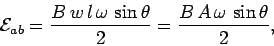(209)

whereis the area of the coil. The emf is zero whenor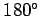, since the direction of motion of sidesandis parallel to the direction of the magnetic field in these cases. The emf attains its maximum value when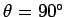or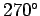, since the direction of motion of sidesandis perpendicular to the direction of the magnetic field in these cases. Incidentally, it is clear, from symmetry, that no net motional emf is generated in sides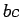and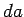of the coil.

Suppose that the direction of rotation of the coil is such that sideis moving into the page in Fig. 38 (side view), whereas sideis moving out of the page. The motional emf induced in sideacts fromto. Likewise, the motional emf induce in sideacts fromto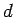. It can be seen that both emfs act in the clockwise direction around the coil. Thus, the net emf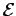acting around the coil is. If the coil hasturns then the net emf becomes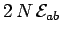. Thus, the general expression for the emf generated around a steadily rotating, multi-turn coil in a uniform magnetic field is(210)

where we have writtenfor a steadily rotating coil (assuming thatat). This expression can also be written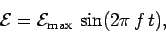(211)

where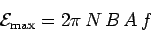(212)

is the peak emf produced by the generator, and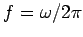is the number of complete rotations the coils executes per second. Thus, the peak emf is directly proportional to the area of the coil, the number of turns in the coil, the rotation frequency of the coil, and the magnetic field-strength.

Figure 39 shows the emf specified in Eq. (211) plotted as a function of time. It can be seen that the variation of the emf with time is sinusoidal in nature. The emf attains its peak values when the plane of the coil is parallel to the plane of the magnetic field, passes through zero when the plane of the coil is perpendicular to the magnetic field, and reverses sign every half period of revolution of the coil. The emf is periodic (i.e., it continually repeats the same pattern in time), with period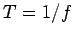(which is, of course, the rotation period of the coil).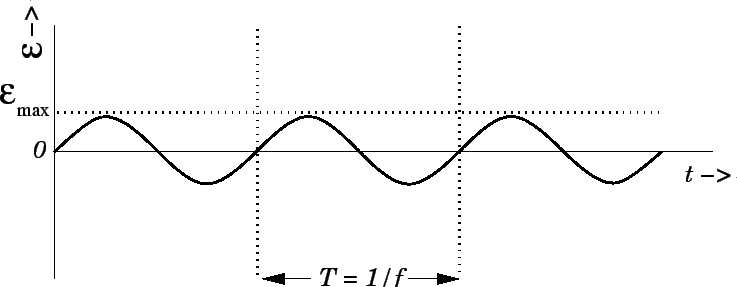Suppose that some load (e.g., a light-bulb, or an electric heating element) of resistanceis connected across the terminals of the generator. In practice, this is achieved by connecting the two ends of the coil to rotating rings which are then connected to the external circuit by means of metal brushes. According to Ohm's law, the current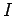which flows in the load is given by(213)

Note that this current is constantly changing direction, just like the emf of the generator. Hence, the type of generator described above is usually termed an alternating current, or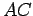, generator.

The currentwhich flows through the load must also flow around the coil. Since the coil is situated in a magnetic field, this current gives rise to a torque on the coil which, as is easily demonstrated, acts to slow down its rotation. According to Sect. 8.11, the braking torque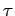acting on the coil is given by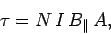(214)

where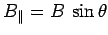is the component of the magnetic field which lies in the plane of the coil. It follows from Eq. (210) that(215)

since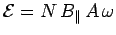. An external torque which is equal and opposite to the breaking torque must be applied to the coil if it is to rotate uniformly, as assumed above. The rate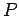at which this external torque does work is equal to the product of the torqueand the angular velocityof the coil. Thus,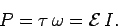(216)

Not surprisingly, the rate at which the external torque performs works exactly matches the rate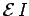at which electrical energy is generated in the circuit comprising the rotating coil and the load.

Equations (210), (213), and (215) yield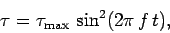(217)

where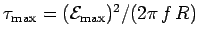. Figure 40 shows the breaking torqueplotted as a function of time, according to Eq. (217). It can be seen that the torque is always of the same sign (i.e., it always acts in the same direction, so as to continually oppose the rotation of the coil), but is not constant in time. Instead, it pulsates periodically with period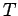. The breaking torque attains its maximum value whenever the plane of the coil is parallel to the plane of the magnetic field, and is zero whenever the plane of the coil is perpendicular to the magnetic field. It is clear that the external torque needed to keep the coil rotating at a constant angular velocity must also pulsate in time with period. A constant external torque would give rise to a non-uniformly rotating coil, and, hence, to an alternating emf which varies with time in a more complicated manner than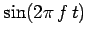.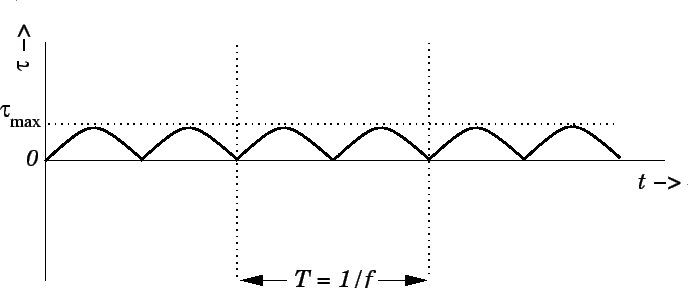Virtually all commercial power stations generate electricity using AC generators. The external power needed to turn the generating coil is usually supplied by a steam turbine (steam blasting against fan-like blades which are forced into rotation). Water is vaporized to produce high pressure steam by burning coal, or by using the energy released inside a nuclear reactor. Of course, in hydroelectric power stations, the power needed to turn the generator coil is supplied by a water turbine (which is similar to a steam turbine, except that falling water plays the role of the steam). Recently, a new type of power station has been developed in which the power needed to rotate the generating coil is supplied by a gas turbine (basically, a large jet engine which burns natural gas). In the United States and Canada, the alternating emf generated by power stations oscillates at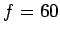Hz, which means that the generator coils in power stations rotate exactly sixty times a second. In Europe, and much of the rest of the world, the oscillation frequency of commercially generated electricity is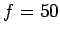Hz.Next: The Direct Current Generator Up: Magnetic Induction Previous: Eddy Currents
Richard Fitzpatrick 2007-07-14